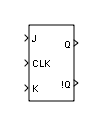# J-K Flip-Flop

Model a negative-edge-triggered J-K flip-flop

• Library:
• Simulink Extras / Flip Flops

•## Description

The J-K Flip-Flop block models a negative-edge-triggered J-K flip-flop. The J-K flip-flop block has three inputs, J, K, and CLK. On the negative (falling) edge of the clock signal (CLK), the J-K Flip-Flop block outputs Q and its complement, !Q, according to the following truth table. In this truth table, Qn-1 is the output at the previous time step.

Note

The J-K Flip-Flop block treats a nonzero input as true (`1`).

JK Q n !Q n
00 Q n-1 !Q n-1
0101
1010
11 !Q n-1 Q n-1

When J is 1 and K is 0, the flip-flop goes to the set state (Qn is 1). When J is 0 and K is 1, the flip-flop goes to the reset state (Qn is 0). When both J and K are 0, the flip-flop stays in the previous state (Qn is Qn-1). When both J and K are 1, the flip-flop toggles (Qn is the complement of Qn-1).

### Logic Signals as Boolean or Double Data Types

The Implement logic signals as boolean data (vs. double) configuration parameter setting affects the input and output data types of the J-K Flip-Flop block because this block is a masked subsystem that uses the Combinatorial Logic block. For more information about this configuration parameter, see Implement logic signals as Boolean data (vs. double).

## Ports

### Input

expand all

Input signal J specified as a scalar, vector, or matrix.

#### Dependencies

The data types that the J-K Flip-Flop block accepts depend on the setting of the Implement logic signals as boolean data (vs. double) configuration parameter:

• `On`J-K Flip-Flop block accepts only real scalar signals of type `boolean`.

• `Off`J-K Flip-Flop block accepts real scalar signals of type `boolean` or `double` (both inputs must have the same type).

Data Types: `double` | `Boolean`

Clock signal, specified as a scalar, vector, or matrix.

#### Dependencies

The data types that the J-K Flip-Flop block accepts depend on the setting of the Implement logic signals as boolean data (vs. double) configuration parameter:

• `On`J-K Flip-Flop block accepts only real scalar signals of type `boolean`

• `Off`J-K Flip-Flop block accepts real scalar signals of type `boolean` or `double` (both inputs must have the same type).

Data Types: `double` | `Boolean`

Input signal K specified as a scalar, vector, or matrix.

#### Dependencies

The data types that the J-K Flip-Flop block accepts depend on the setting of the Implement logic signals as boolean data (vs. double) configuration parameter:

• `On`J-K Flip-Flop block accepts only real scalar signals of type `boolean`

• `Off`J-K Flip-Flop block accepts real scalar signals of type `boolean` or `double` (both inputs must have the same type).

Data Types: `double` | `Boolean`

### Output

expand all

Output signal !Q, with the same dimensions and data type as the input.

Data Types: `double` | `Boolean`

Output signal !Q, with the same dimensions and data type as the input.

Data Types: `double` | `Boolean`

## Parameters

expand all

Specify the initial value of the output Q. A nonzero value is treated as true (`1`).

#### Programmatic Use

 Block Parameter: `initial_condition` Type: character vector Values: scalar Default: `'0'`

## Version History

Introduced in R2008b